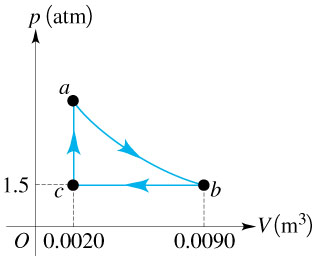# Problem: The figure below shows the PV cycle for a heat engine that uses 2 moles of a monoatomic gas. The initial temperature is Ta = 300 K. The engine runs through 3 process: an isotherm, an isobar, and an isochore.(a) Find explicit values of the gas variables P, V, and T at all 3 points on the diagram(b) Calculate ΔU, W, and Q for each leg of the cycle(c) What is the work done by the engine in one cycle?(d) What is the engine's thermal efficiency?

###### FREE Expert Solution
89% (471 ratings)
###### Problem Details

The figure below shows the PV cycle for a heat engine that uses 2 moles of a monoatomic gas. The initial temperature is Ta = 300 K. The engine runs through 3 process: an isotherm, an isobar, and an isochore.

(a) Find explicit values of the gas variables P, V, and T at all 3 points on the diagram

(b) Calculate ΔU, W, and Q for each leg of the cycle

(c) What is the work done by the engine in one cycle?

(d) What is the engine's thermal efficiency?